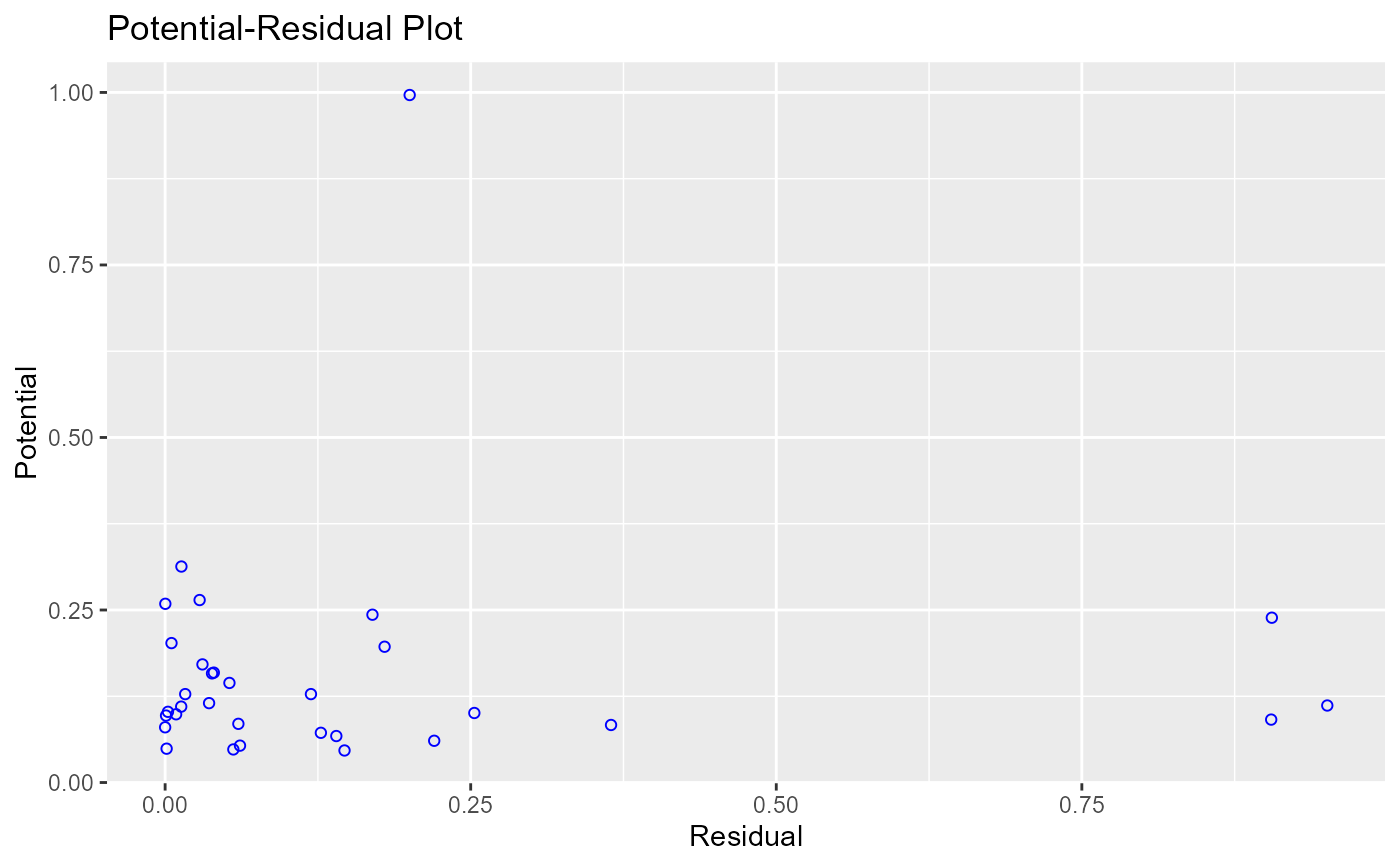Plot to aid in classifying unusual observations as high-leverage points, outliers, or a combination of both.

ols_plot_resid_pot(model, print_plot = TRUE)

## Arguments

model An object of class lm. logical; if TRUE, prints the plot else returns a plot object.

## Deprecated Function

ols_potrsd_plot() has been deprecated. Instead use ols_plot_resid_pot().

Chatterjee, Samprit and Hadi, Ali. Regression Analysis by Example. 5th ed. N.p.: John Wiley & Sons, 2012. Print.

model <- lm(mpg ~ disp + hp + wt, data = mtcars)
ols_plot_resid_pot(model)